# reactance

Also found in: Dictionary, Thesaurus, Medical, Acronyms, Wikipedia.

## impedance

impedance, in electricity, measure in ohms of the degree to which an electric circuit resists the flow of electric current when a voltage is impressed across its terminals. Impedance is expressed as the ratio of the voltage impressed across a pair of terminals to the current flow between those terminals. When a circuit is supplied with steady direct current, the impedance equals the total resistance of the circuit. The resistance depends upon the number of electrons that are free to become part of the current and upon the difficulty that the electrons have in moving through the circuit. When a circuit is supplied with alternating current, the impedance is affected by the inductance and capacitance in the circuit. When supplied with alternating current, elements of the circuit that contain inductance or capacitance build up voltages that act in opposition to the flow of current. This opposition is called reactance, and it must be combined with the resistance to find the impedance. The reactance produced by inductance is proportional to the frequency of the alternating current. The reactance produced by capacitance is inversely proportional to the frequency of the alternating current. In order for a source of electricity that has an internal impedance to transfer maximum power to a device that also has an impedance, the two impedances must be matched. For example, in the simple case of pure resistances, the resistance of the source must also equal the resistance of the device. Impedance matching is important in any electrical or electronic system in which power transfer must be maximized.

## Reactance

The imaginary part of the impedance of an alternating-current circuit.

The impedance Z of an alternating current circuit is a complex number given by Eq. (1).

(1)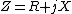(2)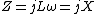(3)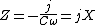The imaginary part X is the reactance. The units of reactance, like those of impedance, are ohms. Reactance may be positive or negative. For example, the impedance of an inductor L at frequency &ohgr; is given by Eq. (2), so X is positive. The impedance of a capacitor C is given by Eq. (3), so X is negative.

The reactance of a circuit may depend on both the resistors and the inductors or capacitors in the circuit. For example, the circuit in the illustration has admittance [Eq. (4)] and impedance [Eq. (5)], so that the reactance [Eq. (6)], depends on both the capacitor C and the resistor R. (4)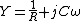(5)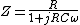(6)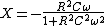See Admittance, Electrical impedance

McGraw-Hill Concise Encyclopedia of Physics. © 2002 by The McGraw-Hill Companies, Inc.
The following article is from The Great Soviet Encyclopedia (1979). It might be outdated or ideologically biased.

## Reactance

in electricity, a quantity characterizing the opposition presented to an alternating current by the capacitance and inductance of a circuit or part of a circuit. Reactance is measured in ohms.

In the case of a sinusoidal current in a circuit where inductive and capacitive circuit elements are connected in series, the reactance x can be expressed as the difference between the inductive and capacitive reactances: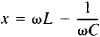Here, to is the angular frequency of the current, L is the inductance of the circuit, and C is the capacitance of the circuit. Reactance is equal to the ratio of the amplitude of the voltage on the terminals of a circuit having little resistance and the amplitude of the current through the circuit. When an alternating current flows in a circuit having only reactance, energy is transferred from the current source to the electric or magnetic field produced, respectively, by the capacitive or inductive circuit element and then back to the current source; the average power during a period is equal to zero. The presence of reactance in a circuit causes a phase difference between the voltage and the current.

When the current in a circuit is nonsinusoidal, the reactance is different for the individual harmonic components of the current.

## reactance

[rē′ak·təns]
(electricity)
The imaginary part of the impedance of an alternating-current circuit.
McGraw-Hill Dictionary of Scientific & Technical Terms, 6E, Copyright © 2003 by The McGraw-Hill Companies, Inc.

## reactance

1. the opposition to the flow of alternating current by the capacitance or inductance of an electrical circuit; the imaginary part of the impedance Z, Z = R + iX, where R is the resistance, i = &#221A--1, and X is the reactance. It is expressed in ohms
2. the opposition to the flow of an acoustic or mechanical vibration, usually due to inertia or stiffness. It is the magnitude of the imaginary part of the acoustic or mechanical impedance
Collins Discovery Encyclopedia, 1st edition © HarperCollins Publishers 2005
References in periodicals archive ?
The finding involving participation in decision-making having a significantly positive relationship with workplace deviance was not expected as reactance theory anticipated a negative relationship.
XrsSD: average reactance with standard deviation; RrsSD: average resistance with standard deviation; 'significant differences (ANOVA test) for p < 0.05; AK: patients with controlled asthma; ANK: patients with uncontrolled asthma; K: control group.
(a) Power transmission efficiency versus load reactance. (b) Output power versus load reactance.
Thus, we conclude that the resistance and reactance parameters of IOS may be useful in clinical settings.
Estimated Equivalent Circuit Parameters Parameter Value, ohm Stator winding resistance [R.sub.1] 0.276 Stator leakage reactance [X.sub.1] 1.56 Magnetizing reactance [X.sub.m] 21.9 Core loss resistance [R.sub.c] 722 Rotor winding resistance [R.sub.2] 0.207 Rotor leakage reactance 1.05
Such assessment provides possibility in each case to take into account (or ignore) the mass reactance forces effect onto fluctuations in the fluid of the given material object.
Convergent validity was examined by calculating the correlation between the GSE with health locus of control, psychological reactance, and attitude towards drug treatment.
This may even lead to a psychological reactance response--a negative reaction toward persuasion attempts (Brehm and Brehm 1981).

Site: Follow: Share:
Open / Close